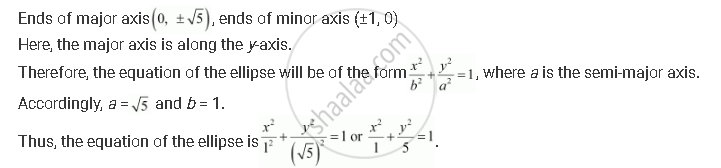# Find the Equation for the Ellipse that Satisfies the Given Conditions: Ends of Major Axis (0, +- Sqrt5), Ends of Minor Axis (±1, 0) - Mathematics

Find the equation for the ellipse that satisfies the given conditions: Ends of major axis (0, +- sqrt5), ends of minor axis (±1, 0)

#### SolutionConcept: Ellipse - Standard Equations of an Ellipse
Is there an error in this question or solution?

#### APPEARS IN

NCERT Class 11 Mathematics
Chapter 11 Conic Sections
Q 14 | Page 255